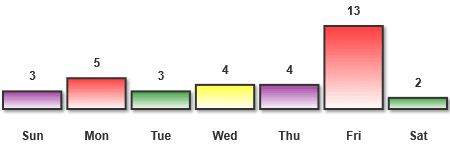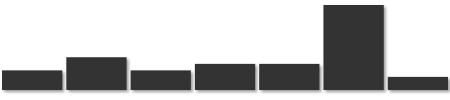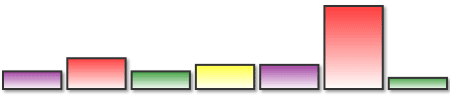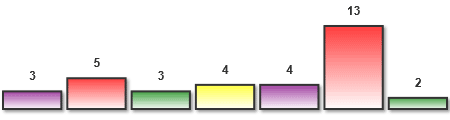# Create a Bar Graph with HTML5 Canvas and JavaScript

This tutorial will show how to create a simple bar graph on an HTML5 Canvas using JavaScript. The code is available at the end of the article for download.

The example below represents the number of sightings of watermelon duck by region updated each second.

Watermelon Duck Sightings by Region

First we will look at the bar graph and what it can do, followed by an example of the graph in action. Then we go step by step through the code and learn how it was done. You can download the source code at the end of the article.

## Introducing the HTML5 Bar Graph

The bar graph we are going to create displays a list of rectangles of varying height proportional to the values they represent. The number of bars, their values and labels are all inputs. If the values change the heights will animate from the old to the new value.

The bar graph has many properties that describe how the data is displayed including color, margin and speed. Many of these properties are configurable including:

 width The total width of the graph height The total height of the graph including the x-axis labels (if included) colors An array of colors (e.g. ["red", "white", "#0000FF"]). If the number of bars is greater than the number of colors the colors will repeat margin The amount of space in pixels required between bars backgroundColor The color behind everything maxValue The maximum value. If this value is not defined then the heights will all be proportional to the largest value of all the current values

## Usage Example

Let's look at a practical example. If I wanted to show a graph representing my car horn behavior last week this is what the graph looks like (Friday was a bad day):

Number of Times I Honked My Horn This WeekThe markup contains a few key items: the canvas where the graph will be drawn, the bar graph script (barGraph.js) and the custom script that describes the graph. If you want support for Internet Explorer you need to add the ExplorerCanvas script (excanvas.js).

```<!DOCTYPE html>
<html>
<title>HTML5 Bar Graph</title>
<body>
<canvas id="canvasId"></canvas>
<!--[if IE]><script src="excanvas.js"></script><![endif]-->
<script src="barGraph.js"></script>
<script>
// ... MY CODE ...
</script>
</body>
</html>```

In between those script tags with the "... MY CODE..." comment we add some code create a new bar graph with the canvas's context as the only parameter. We set a few of its properties like margin and dimensions and update the graph with the values we want to display.

```var cxt = document.getElementById("canvasId").getContext("2d");

var graph = new BarGraph(ctx);
graph.margin = 2;
graph.width = 450;
graph.height = 150;
graph.xAxisLabelArr = ["Sun", "Mon", "Tue", "Wed", "Thu", "Fri", "Sat"];
graph.update([3, 5, 3, 4, 4, 13, 2]);```

## The Code Explained

Next we take a look at the code. If you just want to use the code you can download the source code at the bottom. If you want to learn strap in and here we go...

## The BarGraph Object

The BarGraph function takes one parameter called "ctx" which corresponds to the context of the canvas on which to draw the bar graph. In addition to the context parameter the function has private and public variables and methods. Let's start with pieces of the BarGraph we want to keep private.

```function BarGraph(ctx) {

// Private properties and methods

var that = this;
var startArr;
var endArr;
var looping = false;

// Loop method adjusts the height of bar and redraws if neccessary
var loop = function () {
...
};

// Draw method updates the canvas with the current display
var draw = function (arr) {
...
};```

First we declare a variable called "that" which is assigned to the "this" parameter that will be used to access the BarGraph object from inside the functions we will create in a bit. The variables "startArr" and and "endArr" will be used to store the array of values from during animation. The "looping" variable will be used as a boolean to store if the bar graph is currently animating or not.

Next we declare a couple of functions called loop and draw. The loop function that will be used in animation. The draw function is declared that will be used to redraw the graph.

We also create some variables and functions that are public that can be used to update the graph. Note to make these public we augment the "this" parameter instead of declaring with the "var" keyword.

```  // Public properties and methods

this.width = 300;
this.height = 150;
this.maxValue;
this.margin = 5;
this.colors = ["purple", "red", "green", "yellow"];
this.curArr = [];
this.backgroundColor = "#fff";
this.xAxisLabelArr = [];
this.yAxisLabelArr = [];
this.animationInterval = 100;
this.animationSteps = 10;

// Update method sets the end bar array and starts the animation
this.update = function (newArr) {
...
};
}```

## The Draw Method

The draw method updates the canvas with the bar values specified by the array parameter called "arr". This function is private and cannot be invoked directly however it is called internally multiple times while animating from one value to another.

```var draw = function (arr) {

var numOfBars = arr.length;
var barWidth;
var barHeight;
var border = 2;
var ratio;
var maxBarHeight;
var largestValue;
var graphAreaX = 0;
var graphAreaY = 0;
var graphAreaWidth = that.width;
var graphAreaHeight = that.height;
var i;```

We declare several variables that will be used inside the function. In addition we set the initial value of a few of the variables such as "numOfBars" to the the array length, the graph area position to zero and it graph area dimensions to the width and height of the graph object.

```  // Update the dimensions of the canvas only if they have changed
if (ctx.canvas.width !== that.width || ctx.canvas.height !== that.height) {
ctx.canvas.width = that.width;
ctx.canvas.height = that.height;
}

// Draw the background color
ctx.fillStyle = that.backgroundColor;
ctx.fillRect(0, 0, that.width, that.height);

// If x axis labels exist then make room
if (that.xAxisLabelArr.length) {
graphAreaHeight -= 40;
}

// Calculate dimensions of the bar
barWidth = graphAreaWidth / numOfBars - that.margin * 2;
maxBarHeight = graphAreaHeight - 25;

// Determine the largest value in the bar array
var largestValue = 0;
for (i = 0; i < arr.length; i += 1) {
if (arr[i] > largestValue) {
largestValue = arr[i];
}
}```

First we check if the graph dimensions and changed and update them if there is a change. We don't want to set the canvas width or height unless there is a change because it forces a redraw.

Next we fill the background of the graph with the value set by the public variable "backgroundColor". If there are any labels set for the x axis then we subtract some pixels from the "graphAreaHeight" variable to make room.

The bar's size is determined by dividing the graph into equal sections minus the margin between each bar.

Finally the maximum value of the "arr" parameter is determined by looping through and updating the "largestValue" variable when it is bigger.

```  // For each bar
for (i = 0; i < arr.length; i += 1) {
// Set the ratio of current bar compared to the maximum
if (that.maxValue) {
ratio = arr[i] / that.maxValue;
} else {
ratio = arr[i] / largestValue;
}

barHeight = ratio * maxBarHeight;```

Next we iterate through each bar in the array and determine the its height based on the target value and the maximum height. If the "maxValue" property has been set then we use that as the maximum, if not we use the largest value in the parameter array. Using the ratio we set the height of the current bar.

The next step is to draw the background of each bar.```    // Turn on shadow

// Draw bar background
ctx.fillStyle = "#333";
ctx.fillRect(that.margin + i * that.width / numOfBars,
graphAreaHeight - barHeight,
barWidth,
barHeight);

To set the shadow we set a value to the canvas' context's shadow properties something other than zero. In this case we set the color to a light gray (#999) with offset and blur of two pixels. We draw the bar background rectangle with the context's "fillRect" method with the dark gray border color ("#333").

To prevent the next objects from having a shadow we set the shadow properties to zero.

```    // Create gradient

To create the bar gradient we use the "createLinearGradient" method to set the gradient height. The "addColorStop" method defines the percentage of the height where each color starts as well as the destination color.```    ctx.fillStyle = gradient;
ctx.fillRect(that.margin + i * that.width / numOfBars + border,
graphAreaHeight - barHeight + border,
barWidth - border * 2,
barHeight - border * 2);```

Fill the bar with the gradient with the "fillRect" method.

We also want to display a text label on top of each bar. Note this is not currently supported in Internet Explorer.```    // Write bar value
ctx.fillStyle = "#333";
ctx.font = "bold 12px sans-serif";
ctx.textAlign = "center";
// Use try / catch to stop IE 8 from going to error town
try {
ctx.fillText(parseInt(arr[i],10),
i * that.width / numOfBars + (that.width / numOfBars) / 2,
graphAreaHeight - barHeight - 10);
} catch (ex) {}```

To draw the bar value text on top of the bar we set the context's "font" property and then call the "fillText" property with the current bar's value.

Lastly we want to add the label under the X axis if it was specified.```    // Draw bar label if it exists
if (that.xAxisLabelArr[i]) {
// Use try / catch to stop IE 8 from going to error town
ctx.fillStyle = "#333";
ctx.font = "bold 12px sans-serif";
ctx.textAlign = "center";
try {
ctx.fillText(that.xAxisLabelArr[i],
i * that.width / numOfBars + (that.width / numOfBars) / 2,
that.height - 10);
} catch (ex) {}
}
}
}; // End draw method```

## The Loop Method

The loop method is private like the draw method meaning it cannot be invoked directly. It is called by the update method when a change in bar values is detected. Its responsibilty is to determine the amount each bar should change and then call the draw method to display that change. More simply it is the animator.

```var loop = function () {

var delta;
var animationComplete = true;

// Boolean to prevent update function from looping if already looping
looping = true;```

The local "delta" variable will be used to save the amount each bar is changed in height. The local "animationComplete" variable is initially set to true. The "looping" variable is set to true so the knows the loop function is running and will not call it.

```  // For each bar
for (var i = 0; i < endArr.length; i += 1) {
// Change the current bar height toward its target height
delta = (endArr[i] - startArr[i]) / that.animationSteps;
that.curArr[i] += delta;
// If any change is made then flip a switch
if (delta) {
animationComplete = false;
}
}```

We loop through each bar and set the amount the bar should change based on the start and end array and the amount of frames in the variable "animationSteps". If there is any change then change the "animationComplete" variable so we know we need to continue.

```
// If no change was made to any bars then we are done
if (animationComplete) {
looping = false;
} else {
// Draw and call loop again
draw(that.curArr);
setTimeout(loop, that.animationInterval / that.animationSteps);
}
}; // End loop function```

If no changes were detected then we stop, otherwise we call the "draw" method with the updated graph array and use "setTimeout" to call the "loop" method again.

## The Update Method

To update the bar graph we call the update method with an array of the new values of the bar graph.

```this.update = function (newArr) {

// If length of target and current array is different
if (that.curArr.length !== newArr.length) {
that.curArr = newArr;
draw(newArr);
} else {
// Set the starting array to the current array
startArr = that.curArr;
// Set the target array to the new array
endArr = newArr;
// Animate from the start array to the end array
if (!looping) {
loop();
}
}
}; // End update method```

First thing we check is if the new values are different from the current values. If the values are the same we update the current array with the new array and call the "draw" method to update the view.

If the target array is different from the current array then we update the start and end array variables and call the "loop" method.